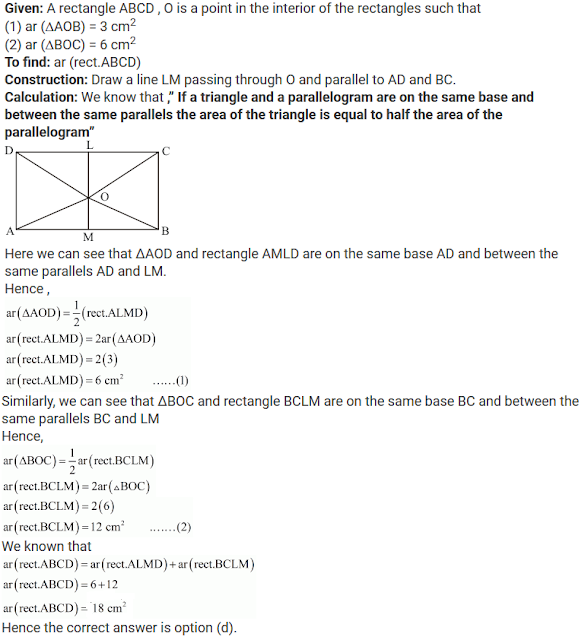#### Chapter 15 Areas of Parallelogram and Triangles R.D. Sharma Solutions for Class 9th MCQ's

Multiple Choice Questions

1. Two parallelograms are on the same base and between the same parallels. The ratio of their areas is
(a) 1 : 2
(b) 2 : 1
(c) 1 : 1
(d) 3 : 1

Solution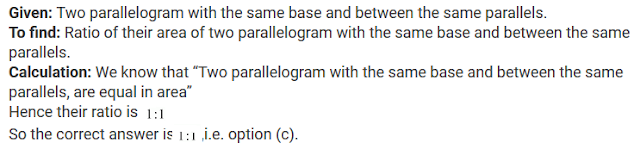2. A triangle and a parallelogram are on the same base and between the same parallels. The ratio of the areas of triangle and parallelogram is
(a) 1 : 1
(b) 1 : 2
(c) 2 : 1
(d) 1 : 3

Solution3. Let ABC be a triangle of area 24 sq. units and PQR be the triangle formed by the mid-points of the sides of Δ ABC. Then the area of ΔPQR is
(a) 12 sq. units
(b) 6 sq. units
(c) 4 sq. units
(d) 3 sq. units

Solution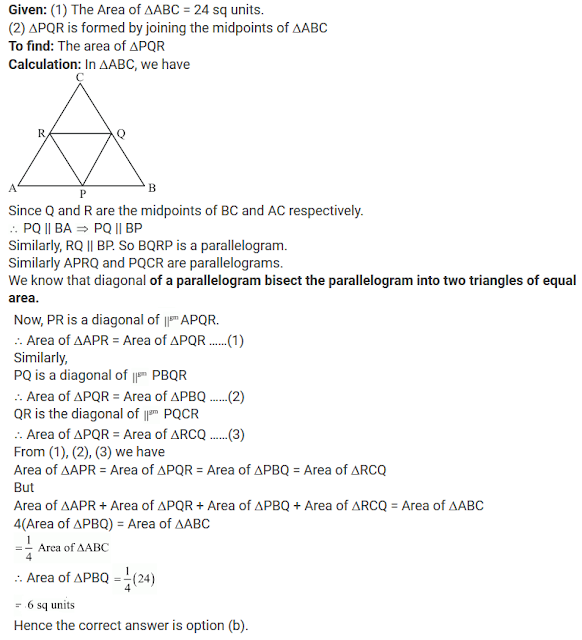4. The median of a triangle divides it into two
(a) congruent triangle
(b) isosceles triangles
(c) right triangles
(d) triangles of equal areas

Solution

Given: A triangle with a median.
Calculation: We know that a ,”median of a triangle divides it into two triangles of equal area.”
Hence the correct answer is option (d).

5. In a △ABC, D, E, F are the mid-points of sides BC, CA and respectively. If ar(△ABC) = 16cm2, then ar(trapezium FBCE) =
(a) 4 cm2
(b) 8 cm2
(c) 12 cm2
(d) 10 cm2

Solution6. ABCD is a parallelogram. P is ant point on CD. If ar(△DPA) = 10 cm2 and ar(△APC) 20 cm2, then ar(△APB) =
(a) 15 cm2
(b) 20 cm2
(c) 35 cm2
(d) 30 cm2

Solution7. The area of the figure formed by joining the mid-points of the adjacent sides of a rhombus with diagonals 16 cm and 12 cm is:
(a) 28 cm2
(b) 48 cm2
(c) 96 cm2
(d) 24 cm2

Solution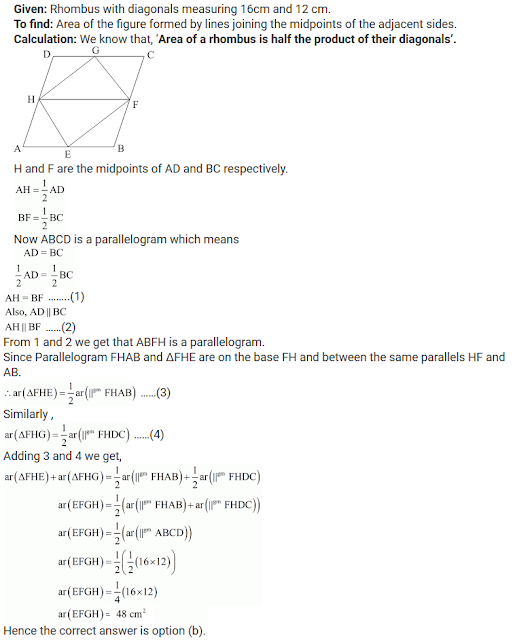8. A, B, C, D are mide-points of sides of parallelogram PQRS. If ar(PQRS) = 36 cm2, then ar(ABCD) =
(a) 24 cm2
(b) 18 cm2
(c) 30 cm2
(d) 36 cm2

Solution9. The figure obtained by joining the mid-points of the adjacent sides of a rectangle of sides 8 cm and 6 cm is:
(a) a rhombus of area 24 cm2
(b) a rectangle of area 24 cm2
(c) a square of area 26 cm2
(d) a trapezium of area 36 cm2

Solution10. The  mid-points of the sides of triangle ABC along with any of the vertices as the fourth point make a parallelogram of area equal to
(a) ar(△ABC)
(b) 1/2 ar(△ABC)
(c) 1/3 ar(△ABC)
(d) 1/4 ar(△ABC)

Solution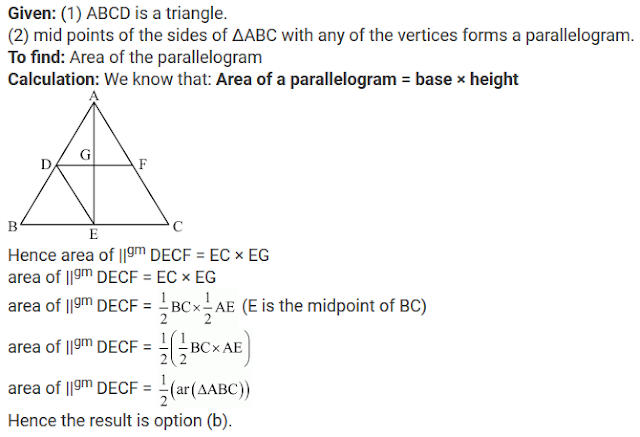11. If AD is median of △ABC and P is a point on AC such that
ar(△ADP) : ar(△ABD) = 2:3, then ar(△PDC) : ar(△ABC)
(a) 1:5
(b) 1:3
(c) 1:6
(d) 3:5

Solution12. Medians of △ABC intersect at G. If ar(△ABC) = cm2, then ar(△BGC) =
(a) 6 cm2
(b) 9 cm2(c) 12 cm2
(d) 18 cm2

Solution13. In a △ABC if D and E are mid-points of BC and AD respectively such that ar(△AEC) = 4 cm2, then ar(△BEC) =
(a) 4 cm2
(b) 6 cm2
(c) 8 cm2
(d) 12 cm2

Solution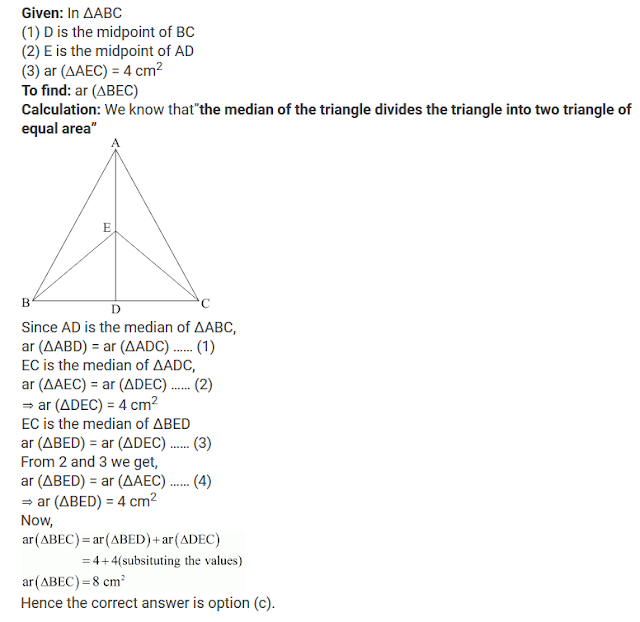14. In Fig. 15.106, ABCD is a parallelogram. If AB = 12 cm, AE = 7.5 cm, CF = 15 cm, then AD =Solution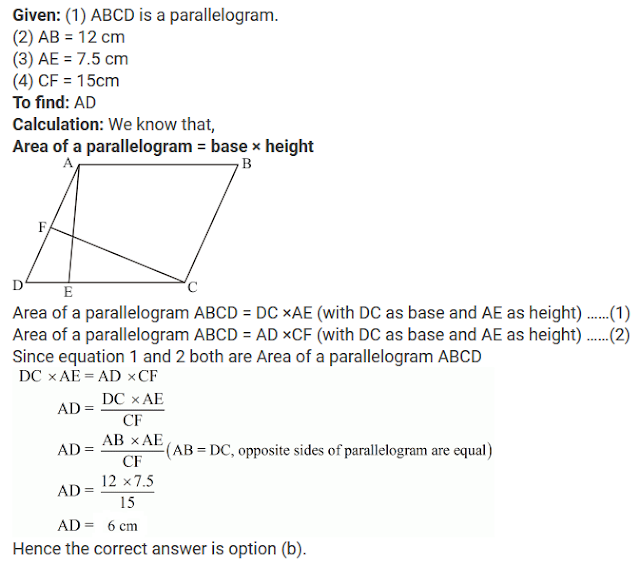15. In Fig. 15.107, PQRS is a parallelogram. If X and Y are mid-points of PQ and SR respectively and diagonal Q is joined. The ratio ar(∥gm XQRY) : ar(△QSR) =
(i) 1:4
(ii) 2:1
(iii) 1:2
(iv) 1:1Solution16. In Fig. 15.108, ABCD and FECG are parallelograms equal in area. If ar(△AQE) = 12 cm2, then ar(∥gm FGBQ) =
(a) 12 cm2
(b) 20 cm2
(c) 24 cm2
(d) 36 cm2Solution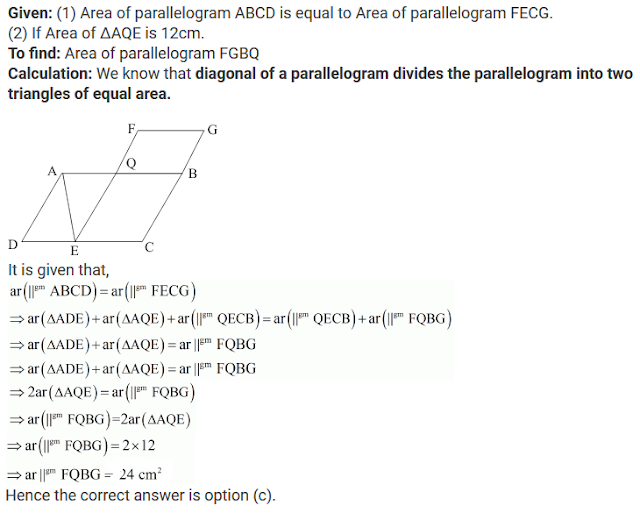17. Diagonal AC and BD of trapezium ABCD, in which AB || DC, intersect each other at O. The triangle which is equal in area of ΔAOD is
(a) ΔAOB
(b) ΔBOC
(c) ΔDOC

Solution18. ABCD is a trapezium in which AB∥DC. If ar(ΔABD) = 24 cm2 and AB = 8 cm, then height of △ABC is
(a) 3 cm
(b) 4 cm
(c) 6 cm
(d) 8 cm

Solution19. ABCD is a trapezium with parallel sides AB =a and DC = b. If E and F are mid-points of non-parallel sides AD and BC respectively, then the ratio of areas of quadrilaterals ABFE and EFCD is
(a) a : b
(b) (a + 3b): (3a + b)
(c) (3a + b) : (a + 3b)
(d) (2a + b) : (3a + b)

Solution20. ABCD is a rectangle with O as any point in its interior. If ar(△AOD) = 3 cm2 and ar(△BOC) = 6 cm2, then area of rectangle ABCD is
(a) 9 cm2
(b) 12 cm2
(c) 15 cm2
(d) 18 cm2

Solution